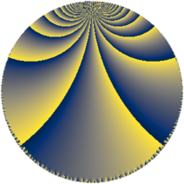# Properties

 Label 384.5.lLevel $384$ Weight $5$ Character orbit 384.l Rep. character $\chi_{384}(31,\cdot)$ Character field $\Q(\zeta_{4})$ Dimension $64$ Newform subspaces $2$ Sturm bound $320$ Trace bound $11$

# Related objects

## Defining parameters

 Level: $$N$$ $$=$$ $$384 = 2^{7} \cdot 3$$ Weight: $$k$$ $$=$$ $$5$$ Character orbit: $$[\chi]$$ $$=$$ 384.l (of order $$4$$ and degree $$2$$) Character conductor: $$\operatorname{cond}(\chi)$$ $$=$$ $$16$$ Character field: $$\Q(i)$$ Newform subspaces: $$2$$ Sturm bound: $$320$$ Trace bound: $$11$$

## Dimensions

The following table gives the dimensions of various subspaces of $$M_{5}(384, [\chi])$$.

Total New Old
Modular forms 544 64 480
Cusp forms 480 64 416
Eisenstein series 64 0 64

## Trace form

 $$64 q + O(q^{10})$$ $$64 q + 3456 q^{29} - 7296 q^{37} + 21952 q^{49} - 1920 q^{53} - 7552 q^{61} + 8064 q^{65} + 19584 q^{69} - 18816 q^{77} - 46656 q^{81} + 22400 q^{85} + O(q^{100})$$

## Decomposition of $$S_{5}^{\mathrm{new}}(384, [\chi])$$ into newform subspaces

Label Dim $A$ Field CM Traces $q$-expansion
$a_{2}$ $a_{3}$ $a_{5}$ $a_{7}$
384.5.l.a $32$ $39.694$ None $$0$$ $$0$$ $$0$$ $$0$$
384.5.l.b $32$ $39.694$ None $$0$$ $$0$$ $$0$$ $$0$$

## Decomposition of $$S_{5}^{\mathrm{old}}(384, [\chi])$$ into lower level spaces

$$S_{5}^{\mathrm{old}}(384, [\chi]) \cong$$ $$S_{5}^{\mathrm{new}}(16, [\chi])$$$$^{\oplus 8}$$$$\oplus$$$$S_{5}^{\mathrm{new}}(48, [\chi])$$$$^{\oplus 4}$$$$\oplus$$$$S_{5}^{\mathrm{new}}(64, [\chi])$$$$^{\oplus 4}$$$$\oplus$$$$S_{5}^{\mathrm{new}}(128, [\chi])$$$$^{\oplus 2}$$$$\oplus$$$$S_{5}^{\mathrm{new}}(192, [\chi])$$$$^{\oplus 2}$$